• 主要介绍了详解C++中十六进制字符串转数字（数值）的相关资料,这里提供两种实现方法，需要的朋友可以参考下
• oracle通过sql实现字符串转数字oracle函数，可用于字符串的最终排序
• 字符串str转数字: float(str) int(str) 数字num转字符串 str(num) a ='1234' print('a = ',a) print(type(a)) b = int(a) print('b = ',b) print(type(b)) c = float(a) print('c = ',c) print...
字符串str转数字:
float(str)int(str)
数字num转字符串
str(num)
a ='1234'
print('a = ',a)
print(type(a))
b = int(a)
print('b = ',b)
print(type(b))
c = float(a)
print('c = ',c)
print(type(c))
d = str(b)
print('d = ',d)
print(type(d))
f = 1.234
ff = str(f)
print('ff = ',ff)
print(type(ff))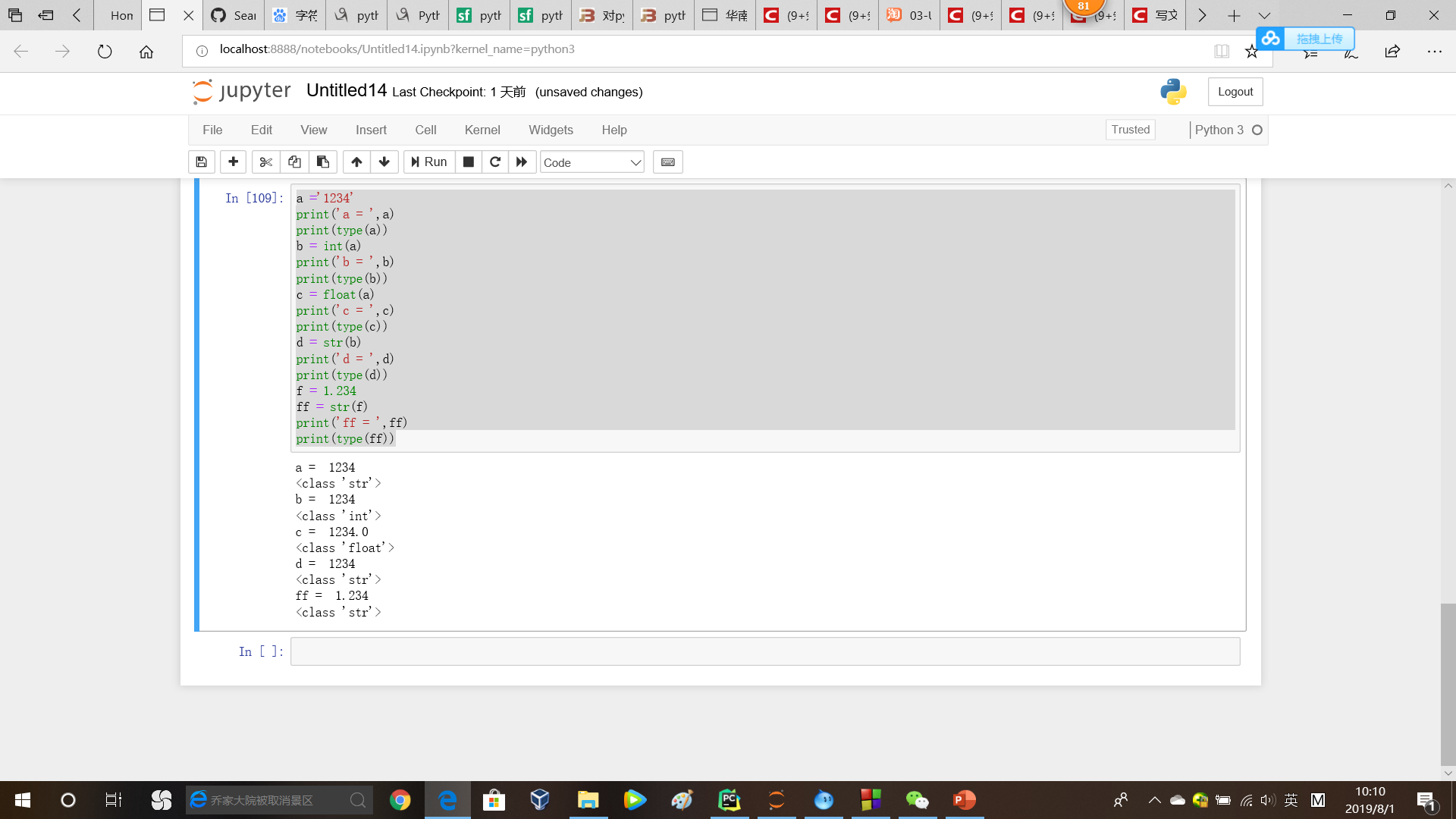数字num转bytes
需将num转为str，再利用codec的encode函数，将str转为bytes：encode(str(num))
bytes转数字
int(bytes)float(bytes)
from codecs import encode, decode

b = b'1.234'
print('b = ',b)
print(type(b))
c = float(b)
print('c = ',c)
print(type(c))
d = str(c)
e = encode(d)
print('e = ',e)
print(type(e))
f = decode(e)
print('f = ',f)
print(type(f))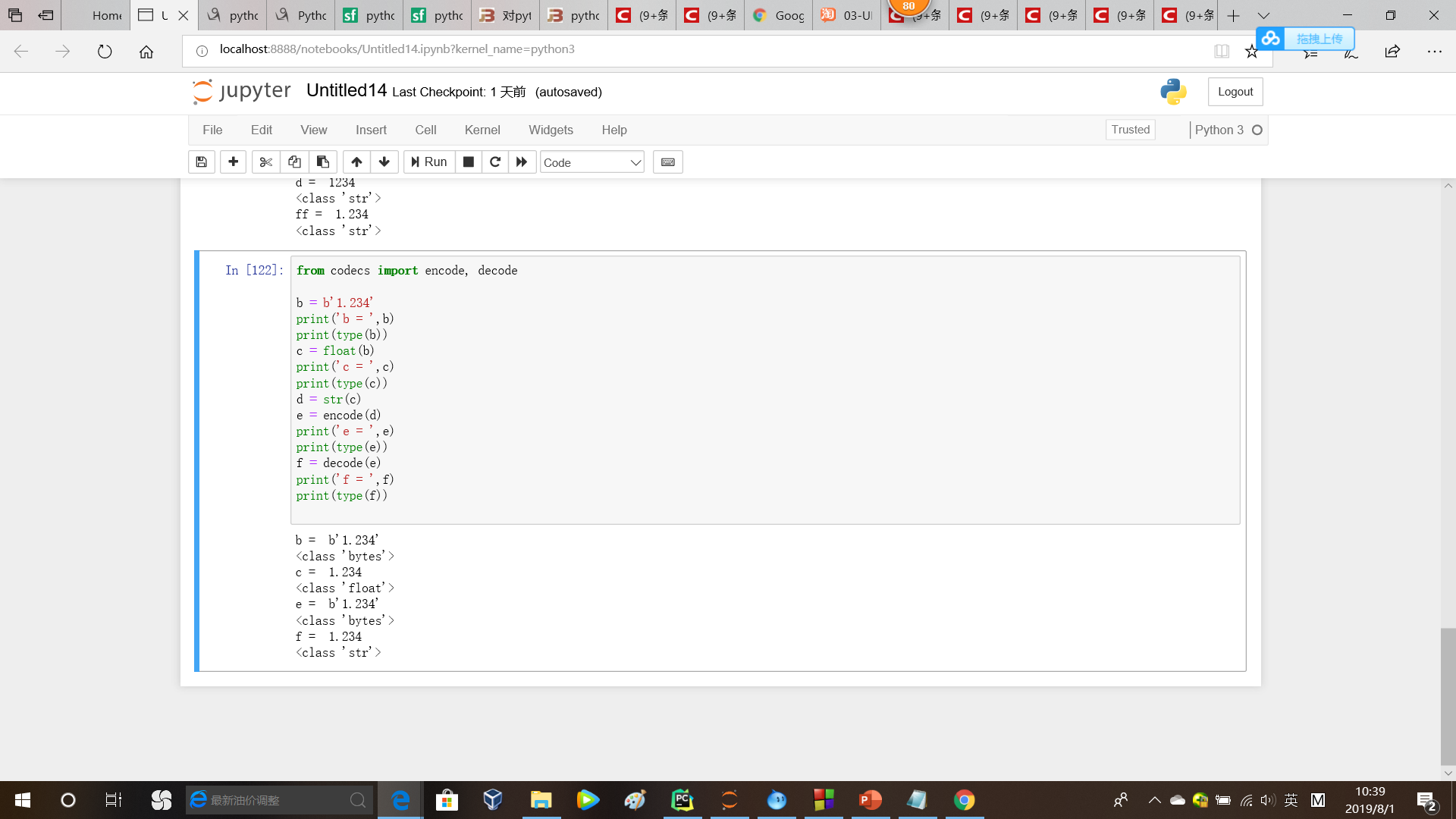字符串转bytes
方法1
from codec import encode,decode
encode(str)

方法2
bytes(str,'UTF-8')

bytes转字符串
方法1
from codec import encode,decode
decode(bytes)

方法2
str(bytes,'UTF-8')

数字表达字符串
cmd ='\x02\x73\x54\x49\x20\x03\x0a'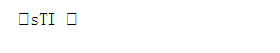综合例子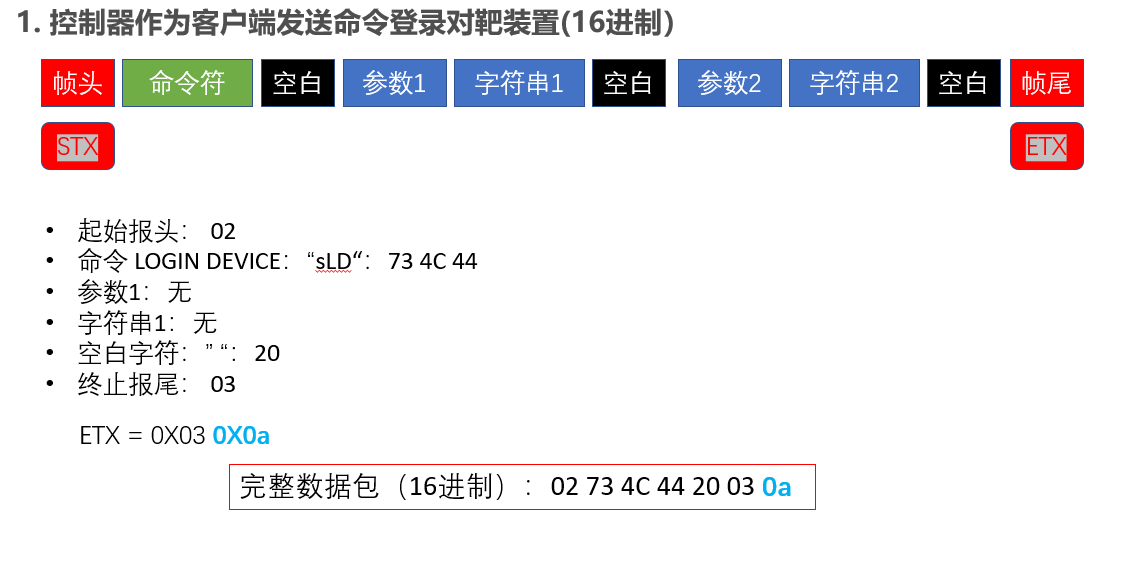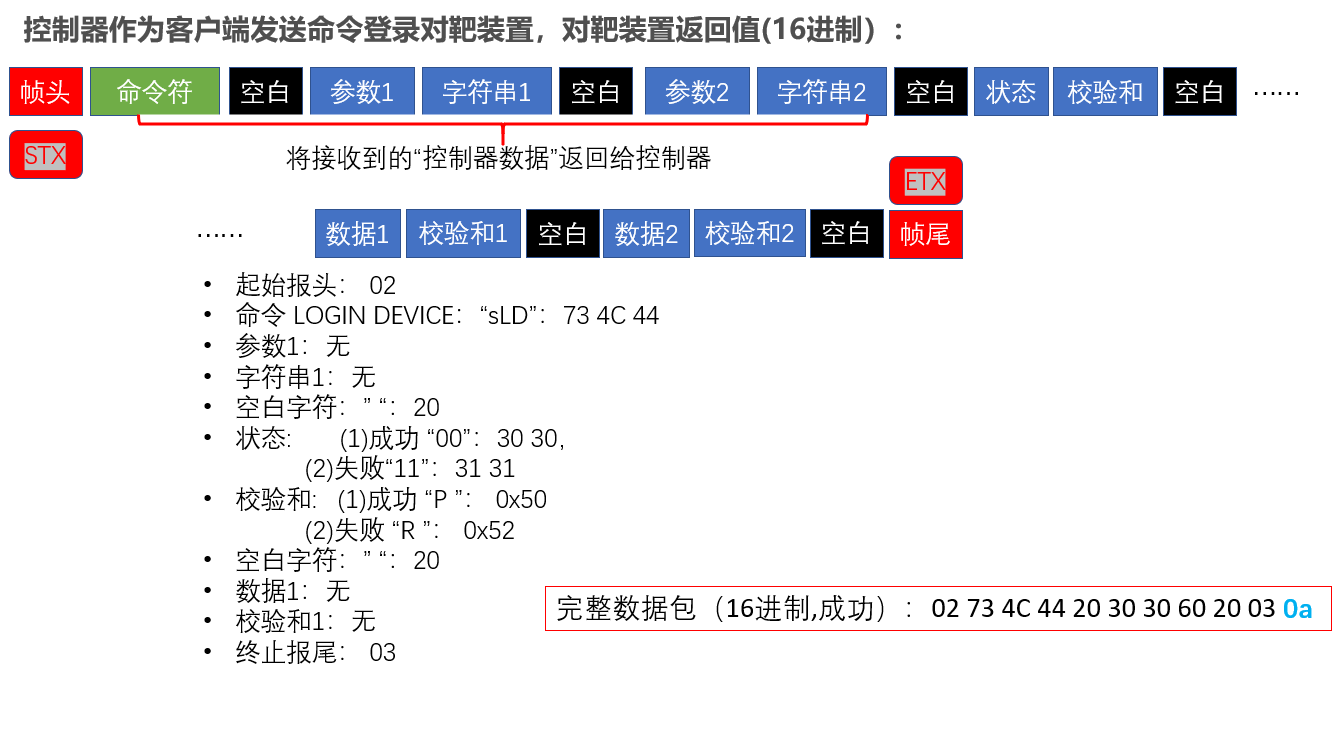SPLIT = '\x20'
LF ='\x0a'

def controller_resp(cmd,statuses,data = None):
'''
cmd: 接收到的控制器命令，用于返回给控制器，字符串
statuses: 对靶装置状态信息，用于返回给控制器，字符串
data: 发送给控制器的数据，用于返回给控制器,注意:data为列表！！里面的数据为str类型！！
'''
data_str = ''
# 生成要发送的数据：数据+校验和+分割符(0x20)
if data != None:
for i in data:
data_str = data_str + i + 'P'+SPLIT #字符串

statuses_part = statuses + 'P'+SPLIT #字符串

#if self.__check_cmd(cmd,self.CONTROLLER_LOGOUT):
#cmd[:-2]表明去掉最后的'\n'
# 命令行+状态部分+ 数据部分
if cmd[-1] == '\n' and cmd[-2] == chr(0x03):
command = cmd[:-2] + statuses_part + data_str + LF
elif cmd[-1] == chr(0x03):
command = cmd[:-1] + statuses_part + data_str + LF

return command #返回命令行字符串
cmd ='\x02\x73\x4c\x44\x20\x03\x0a'
print(cmd)
#cmd = bytes(cmd,'UTF-8')

print(cmd[-1] == '\n')
statuses = '00'

result = controller_resp(cmd,statuses)
print(result)
for i in result:

print(ord(i),i)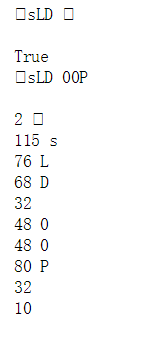展开全文• 字符串转数字 stod()//字符串转double stof()//字符串转float stoi()//字符串转int stol()//字符串转long stold()//字符串转double stoll()//字符串转long long stoul()//字符串转unsigned long stoull()//字符串转...
前言
C++字符串与数字的转换应该是很常见的问题，每次遇到了再去查有点费劲，干脆记录下来
字符串转数字
stod()//字符串转double
stof()//字符串转float
stoi()//字符串转int
stol()//字符串转long
stold()//字符串转double
stoll()//字符串转long long
stoul()//字符串转unsigned long
stoull()//字符串转unsinged long long
//注意！没有unsigned double和unsigned float！！！
//没有 (unsigned+浮点数) 这种类型！！！
//下面用stoi举例，其它类似

结束语
本人大三学生一枚，学识尚浅，不喜勿喷，希望今日能抛砖引玉，请各位大佬一定不吝赐教！！！
展开全文• 之前做个需求遇到字符串转数字，遇到点问题和大家分享下。 我想对查询的结果集分组求和，本以为转换下数据类型用聚合函数直接求和就完事了。就像下面这样 SELECT p.id, p.objno, p.objname, -- bt....
之前做个需求遇到字符串转数字，遇到点问题和大家分享下。
我想对查询的结果集分组求和，本以为转换下数据类型用聚合函数直接求和就完事了。就像下面这样
SELECT
p.id,
p.objno,
p.objname,
-- 	bt.projecttypename,
bic.ratename,
sum(convert(numeric(10,2),bic.ratevalue )),
bic.ratevalue
--CAST ( bic.ratevalue AS NUMERIC(10,2) )

FROM
Biddingproject_approval_budget ba
LEFT JOIN Biddingproject_typemaster bt ON ba.id = bt.tenderid
LEFT JOIN Biddingproject_Interestrate bi ON bi.mastertprojecttypeid = bt.id
LEFT JOIN project p ON p.id = bi.ratevalue
LEFT JOIN Biddingproject_implementation_costs bic ON bic.mastertprojecttypeid = bt.id
LEFT JOIN Biddingproject_typemaster btv ON ba.id = btv.tenderid
WHERE
bi.ratename = '项目子编号ID'
AND bi.ratevalue <> ''
AND bi.ratevalue IS NOT NULL
AND btv.projecttypename = '小计'
AND bt.projecttypename NOT IN ( '小计', '差额' )
AND bic.ratename <> '8、实施费用'
AND ba.id = 'AUAS4IJIUIWLVD3KBOXSLQK9T2NM1148'
GROUP BY
p.id,
bt.id,
bic.ratename,
p.objno,
bic.ratevalue,
p.objname

结果运行转换数据异常。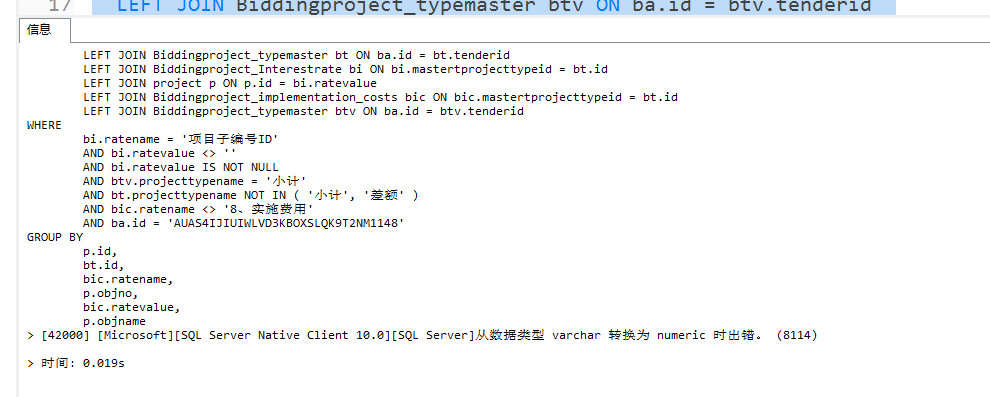想着不应该啊，换 cast  函数  依旧报 数据转换异常。
去掉convert 函数，先看下数据，数据结果如下：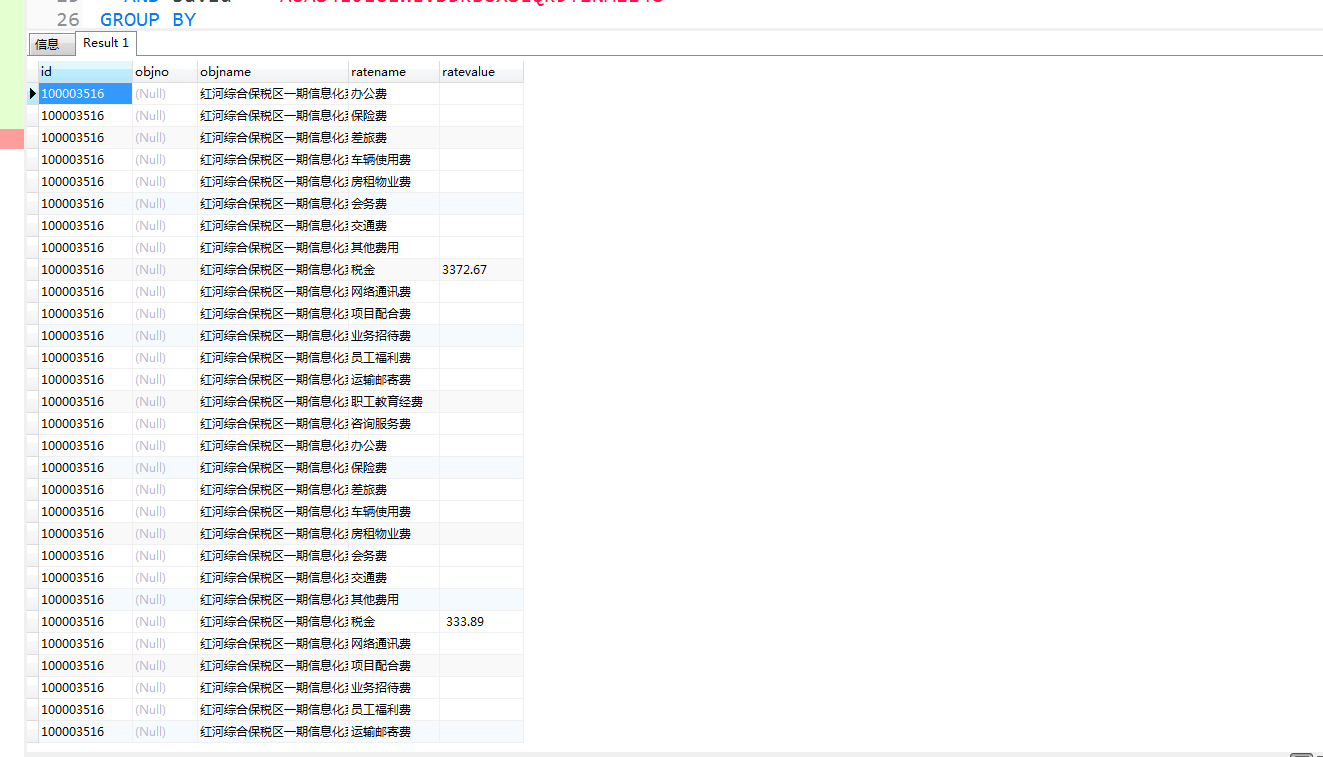原来里面有空字符串，导致无法转换。这时可能有同鞋说用isnull函数 ，然后发现依旧报错。为什么呢？
isnull 函数是对null进行处理的，在这里不起作用。
这里我们要用 case 函数，最终SQL 调整如下
SELECT
p.id,
p.objno,
p.objname,
bic.ratename,
sum(case when bic.ratevalue = '' then 0 else CAST ( bic.ratevalue AS NUMERIC(10,2) ) end )
FROM
Biddingproject_approval_budget ba
LEFT JOIN Biddingproject_typemaster bt ON ba.id = bt.tenderid
LEFT JOIN Biddingproject_Interestrate bi ON bi.mastertprojecttypeid = bt.id
LEFT JOIN project p ON p.id = bi.ratevalue
LEFT JOIN Biddingproject_implementation_costs bic ON bic.mastertprojecttypeid = bt.id
LEFT JOIN Biddingproject_typemaster btv ON ba.id = btv.tenderid
WHERE
bi.ratename = '项目子编号ID'
AND bi.ratevalue <> ''
AND bi.ratevalue IS NOT NULL
AND btv.projecttypename = '小计'
AND bt.projecttypename NOT IN ( '小计', '差额' )
AND bic.ratename <> '8、实施费用'
AND ba.id = 'AUAS4IJIUIWLVD3KBOXSLQK9T2NM1148'
GROUP BY
p.id,
bic.ratename,
p.objno,
p.objname

在这里我们先用case函数把是空的字符串转成 0 ，在这里要注意  case函数 会见数字默认转为 int 类型  这里还需要一点小小的处理。 然后大功告成。结果如下。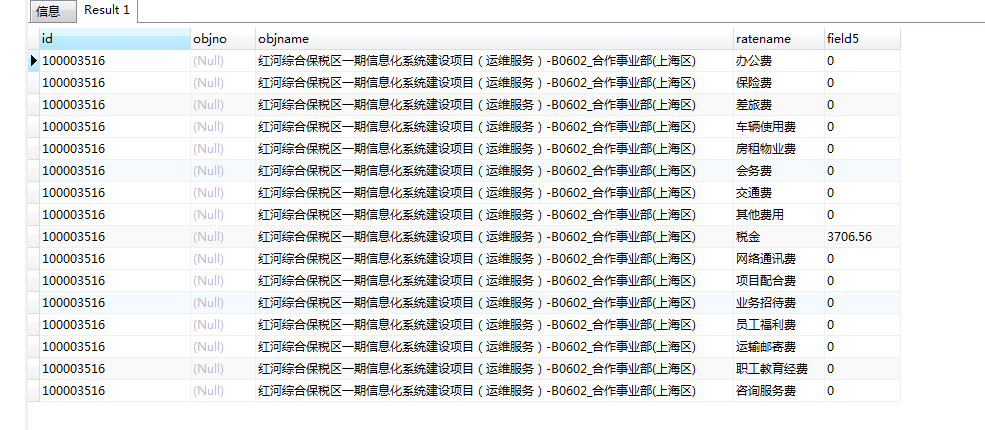展开全文SQL分组求和
• ## 负数字符串转数字

千次阅读 2019-11-14 22:23:58
在用int a = Integer....转数字时报错，“-”无法识别 没找到是否有此类函数直接转换 查询后通过下列语句可以实现 String st=s.next(); String str = null; for(int i=0;i<st.length();i++){ { if (st.ch...
在用int a = Integer.parseInt(str);转数字时报错，“-”无法识别 没找到是否有此类函数直接转换 查询后通过下列语句可以实现
String st=s.next();
String str = null;
for(int i=0;i<st.length();i++){
{
if (st.charAt(i)=='-')
st=st.substring(i+1);
else
str=st;
}}
int a = Integer.parseInt(str);
展开全文• 主要介绍了C语言实现将字符串转换为数字的方法,涉及系统函数atoi()函数的使用技巧,需要的朋友可以参考下
• 12是数字12 12是字符1和字符2 看上去一样的， 实际是不同的 计算机里面，就经常有这样的事情发生 你看上去一样， 还要看看他的本质，是不是一样。 他的源头，是不是一样。 看上去不一样， 其实他的本质又一样。 ...
• 主要介绍了Angular js双向绑定时字符串的转换成数字类型的问题,需要的朋友可以参考下
• 今天小编就为大家分享一篇使用pandas把某一列的字符值转换为数字的实例，具有很好的参考价值，希望对大家有所帮助。一起跟随小编过来看看吧
• C语言数字转字符串 C语言字符串转数字 C语言数字转字符串 C语言提供了几个标准库函数，可以将任意类型(整型、长整型、浮点型等)的数字转换为字符串，下面列举了各函数的方法及其说明。 ● itoa() ：将整型值...
• 今天小编就为大家分享一篇vue 实现强制类型转换 数字类型转为字符串，具有很好的参考价值，希望对大家有所帮助。一起跟随小编过来看看吧
• 1.直接用加法 　字符串字段+0 2.使用函数，这里的type可以为：浮点数 : DECIMAL 、整数 : SIGNED、无符号整数 : UNSIGNED CAST(value as type); CONVERT(value, type);mysql 数据库
• 有效的介绍了西门子PLC字符串转实数功能，让初学者更清晰的了解该功能
• ## js字符串转数字

万次阅读 2019-05-27 15:35:32
• 实现 atoi，将字符串转为整数。 该函数首先根据需要丢弃任意多的空格字符，直到找到第一个非空格字符为止。如果第一个非空字符是正号或负号，选取该符号，并将其与后面尽可能多的连续的数字组合起来，这部分字符即...atoi
• select * from student order by to_number(studentid)排序
• 详解C/C++中十六进制字符串转数字（数值） 主要有两个方法，其实都是对现有函数的使用： 方法1: sscanf() 函数名: sscanf 功 能: 从字符串格式化输入 用 法: int sscanf(char string, char format[,argument,…]); ...
• #字符串转数组，并去除特殊符号，去掉.0转为格式上的整数 list(row.replace('nan','0').replace('.0','').split(',')) ['1', '2', '3','0'] #将格式上的整数，转换为类型上的整数 list(map(int,list(row.replace...python
• 原型： value=$((n#${key}Xm)) ...key:字符串变量 X：操作符；如+ - * / &amp;... m:操作数 实例1：10进制字符32加上32 a='32' value=$((10#${a}+32)) ---------------------------------...Linux Shell
• 利用js变量弱类型转换。将字符串类型转换成数字类型，并执行算术运算 var n = "1"; var str = n-0 ; //此时str已经是数字类型 str = str +1 ; //结果为 str = 2; //也可以写成 var str = n-0 +1;
• 关键代码： sscanf( "0x4988E348AED3C" , "%I64x" , &nValude );关键是 64位的支持 void strTest() { char* p = "0x4988E348AED3C"; char* str; __int64 i = strtol(p, &str,...
• <fmt:formatNumber value="EL表达式" pattern="#.##" type="number" /> 举例： <div style="padding-bottom: 2vw;...fmt:formatNumber value="\${dayIncome.CHECK_COUNTS}" pattern="#.##" type...
• 在C/C++语言中没有专门的字符串变量，通常用字符数组来存放字符串字符串是以“\0”作为结束符。
• 例如： String a = "12.33"; String b = "11.66"; double aa = Double.parseDouble(a); double bb = Double.parseDouble(b);...System.out.println("未转换前："+(bb+aa));...这段代码输出---未转换前：23....java
• 在C/C++语言中没有专门的字符串变量，通常用字符数组来存放字符串字符串是以“\0”作为结束符。C/C++提供了丰富的字符串处理函数，下面列出了几个最常用的函数。  ● 字符串输出函数puts。  ● 字符串输出函数...
• 字符串转数字，需要考虑2点 第一点：字符串为空，需要先将空的字符串为 空、字母、中文、标点等去掉，只能将为数字的转换为数值 ；至于非阿拉伯数字的情况就要业务给出处理方式，下面是一个样例。 select case when...
• ## mfc 字符串转数字

千次阅读 2019-03-31 23:54:31
• .stream().map(AssessRec::getScore).mapToInt(Integer::parseInt).sum() 列表中对象属性是字符串数字，然后汇总...